# Fitting and evaluating a join trait distribution model

In this vignette we explain how to fit, evaluate and interpret a joint trait distribution model (JTDM). We refer to Poggiato et al., in prep. “Joint models and predictions of community traits” for the full description of JTDMs, and we rather focus here on presenting the R package by applying it to the dataset of the case study presented in the publication.

## Installing the R package

## CRAN
#install.packages('jtdm', repos = "http://cran.us.r-project.org")
## Github
#library(devtools)
#install_github("giopogg/jtdm")
library(jtdm)
library(ggplot2)

# Fit a jtdm to data

JTDMs relate a vector of average community traits (e.g. community weighted mean, CWM) to a vector of environmental covariates. The matrix Y contains the CWM traits of specific leaf area (SLA), leaf nitrogen content (LNC) and plant height of 116 plant community sites situated along 21 elevation gradients (www.orchamp.osug.fr) in the French Alps.

data(Y)
summary(Y)
#>       SLA              LNC            Height
#>  Min.   : 3.857   Min.   :14.86   Min.   :10.80
#>  1st Qu.:13.225   1st Qu.:19.71   1st Qu.:23.49
#>  Median :16.349   Median :21.42   Median :35.39
#>  Mean   :20.500   Mean   :22.06   Mean   :34.87
#>  3rd Qu.:21.923   3rd Qu.:23.88   3rd Qu.:44.17
#>  Max.   :66.664   Max.   :34.30   Max.   :76.38

We consider two environmental covariates: Growing Degree Days (GDD, the sum of temperature of days with positive temperature in the growing season) to represent the average length and intensity of the growing season and intensity of freezing events (Freezing Degree Days, the sum of temperature of days with negative temperature in the growing season, FDD). To account for habitat type, we included a variable that was set to 1 when the site was in a forest, 0 otherwise (hereafter habitat). These data are stored in the matrix X.

data(X)
summary(X)
#>       GDD              FDD              forest
#>  Min.   : 467.4   Min.   :-36.838   Min.   :0.0000
#>  1st Qu.:1037.3   1st Qu.:-14.768   1st Qu.:0.0000
#>  Median :1320.7   Median :-11.520   Median :0.0000
#>  Mean   :1357.6   Mean   :-12.758   Mean   :0.3621
#>  3rd Qu.:1684.9   3rd Qu.: -9.461   3rd Qu.:1.0000
#>  Max.   :2861.5   Max.   : -2.522   Max.   :1.0000

JTDMs infer a linear regression for each CWM trait as a function of the environmental covariates, together with an inter-traits residual covariance matrix. Therefore, the parameters of a JTDM are the regression coefficients $$B$$ and the residual covariance $$\Sigma$$. Notice that inferring a generalized linear model is possible, even thus not yet implemented in our package. The inference of JTDM is implemented in the Bayesian framework with the function jtdm_fit. This functions samples from the posterior distribution, which has been analytically determined. Therefore, there is no need for classical MCMC convergence checks. The syntax of the function is similar to the popular function lm. This function requires the response matrix Y, the predictor matrix X and a (right-hand only) formula to specify how CWM traits depends on environmental variables. We choose here to include in the model linear and quadratic terms (using orthogonal polynomials) of GDD and FDD in interaction with habitat to enable non linear trait-environment relationships and to allow for different trait-environment relationships in forest and open habitats. The is very fast, however, to ensure that the whole vignette runs quickly fast, we only sample a relative low number of samples from the posterior distribution (100 here). To obtain reliable results, the number of samples should be increased (around 1000).

# Short MCMC to obtain a fast example: results are unreliable !
m = jtdm_fit(Y = Y, X = X,
formula = as.formula("~poly(GDD,2)+poly(FDD,2)+poly(GDD,2):forest+poly(FDD,2):forest"),
sample = 100)

summary(m)

## Get parameters

Since we ran the JTDM with very short chains, the MCMC has not converged. You can try to run the model with longer chains and check its convergence.

We can now look at the inferred the regression coefficients. The getB function provides the MCMC samples of the regression coefficients matrix $$B$$, together with its mean, and $$95\%$$ credible interval.

# Inferred parameters
B = getB(m)

get_sigma provides instead the MCMC samples of the residual covariance matrix $$\Sigma$$ together with its mean and $$95\%$$ credible interval.

Sigma = get_sigma(m)$Smean We can plot these parameters with the function plot plot(m)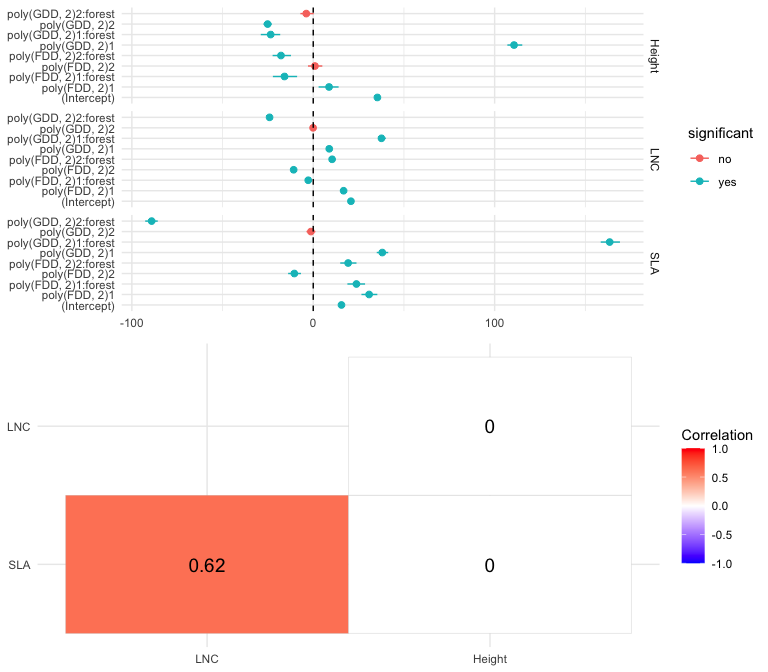## Predict and evaluate model fit We can predict CWM traits on a (new) set of sites using jtdm_predict. Here, we predict on the training dataset and compute goodness of fit measures of these predictions like $$R^2$$ and RMSE, by setting validation=TRUE. We can choose whether to obtain the full posterior predictive distribution (FullPost=TRUE which is more time consuming) or just its posterior mean (FullPost=FALSE, which we advice if the aim is to only compute goodness of fit measures). predictions = jtdm_predict(m, Xnew = X, Ynew = Y,validation = T) predictions$R2
#>       SLA       LNC    Height
#> 0.5881181 0.3524556 0.5215280
predictions$RMSE #> SLA LNC Height #> 7.596181 2.798893 9.126322 We can evaluate the performances of the model using a K-fold cross-validation using the function jtdmCV predictionsCV = jtdmCV(m, K = 5, sample = 100) predictionsCV$R2
predictionsCV$RMSE ## Trait-environment relationships We can now analyze the inferred trait-environment relationships using the function partial_response, that computes and plots the partial response curve of a focal trait along a focal environmental gradient. This function takes as input the model m, the focal environmental variable indexGradient and the focal trait indexTrait, using the names of environmental variables and traits as specified in the column names of X and Y respectively. The function builts a dataset of environmental variables by building the gradient of the focal environmental variable and keeping all other variables fixed to their mean. Then, it predicts the marginal distribution of the focal trait for the so-built environmental dataset. The user can choose whether to obtain the full predictive distribution (FullPost=TRUE), or the predictive distribution of the mean term (FullPost="mean", which we advice here), or just the posterior mean (FullPost="mean"). The function outputs are the plot of the inferred trait-environment relationship and the predictions of the model (used to produce the plot). For example, we can plot the partial response curve relationship between SLA and GDD partial_response(m, indexGradient = "GDD",indexTrait = "SLA")$p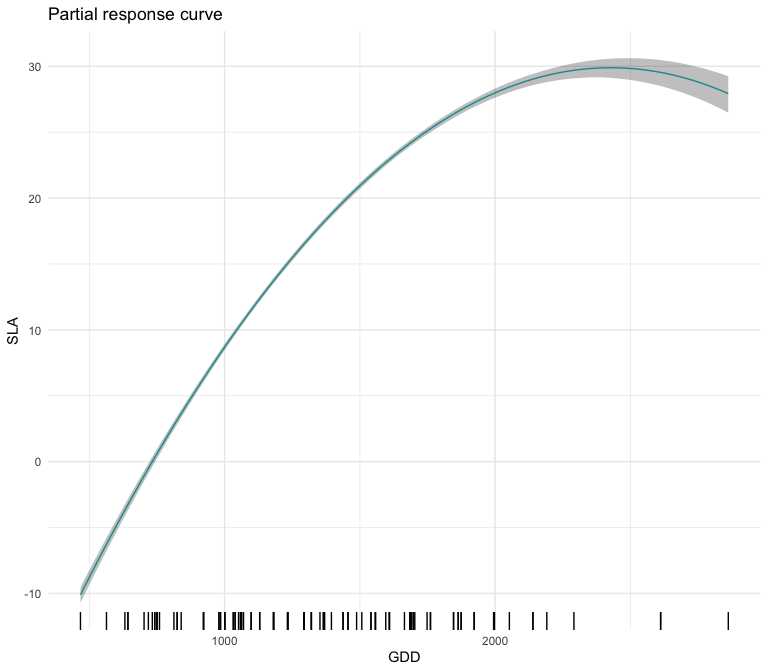The user can eventually provide a given gradient for the focal environmental variable XFocal (which is otherwise built on regular grid with length given by grid.length). The user can also choose to fix the non focal environmental variables to another value. For example, we can obtain the partial response curve of SLA and GDD in forest

partial_response(m, indexGradient = "GDD", indexTrait = "SLA",
FixX = list(GDD = NULL, FDD = NULL, forest = 1))$p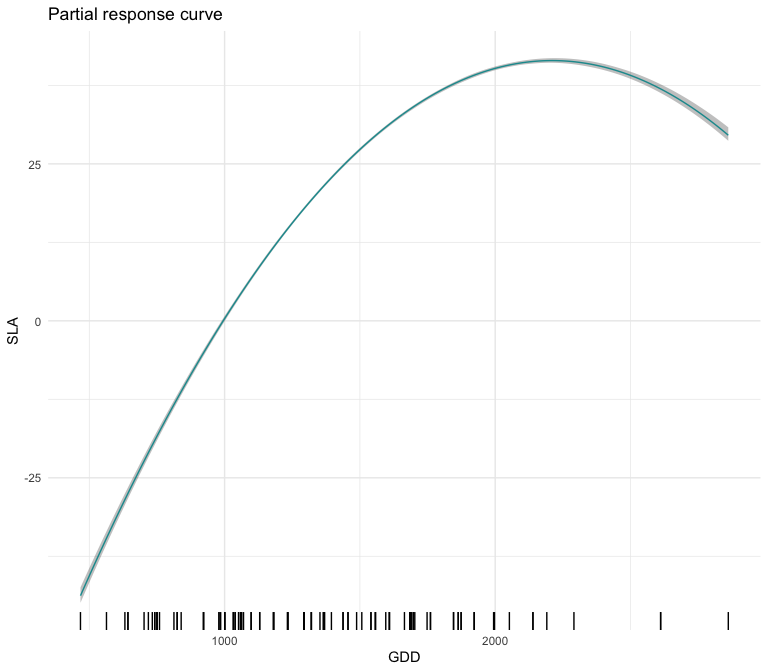## Multivariate confidence intervals We can then compute the partial response curves of pairwise CWM trait combinations together with their $$95\%$$ credible region, what we define in the publication as the most likely CWM combination and envelop of possible CWM combinations. This is done by the function ellipse_plot, that takes as input the model m, the focal environmental variable indexGradient and the two focal traits indexTrait, using the names of environmental variables and traits as specified in the column names of X and Y respectively. The function builds a gradient of the focal environmental variable while keeping all other variables fixed to their mean, and then predict and plots the joint distribution of the focal traits. The user can choose whether to obtain the full predictive distribution (FullPost=TRUE), or the predictive distribution of the mean term (FullPost=F, which we advice here to obtain smoother curves). # plot the pairwise SLA-LNC partial response curve along the GDD gradient ellipse_plot(m, indexTrait = c("SLA","LNC"), indexGradient = "GDD")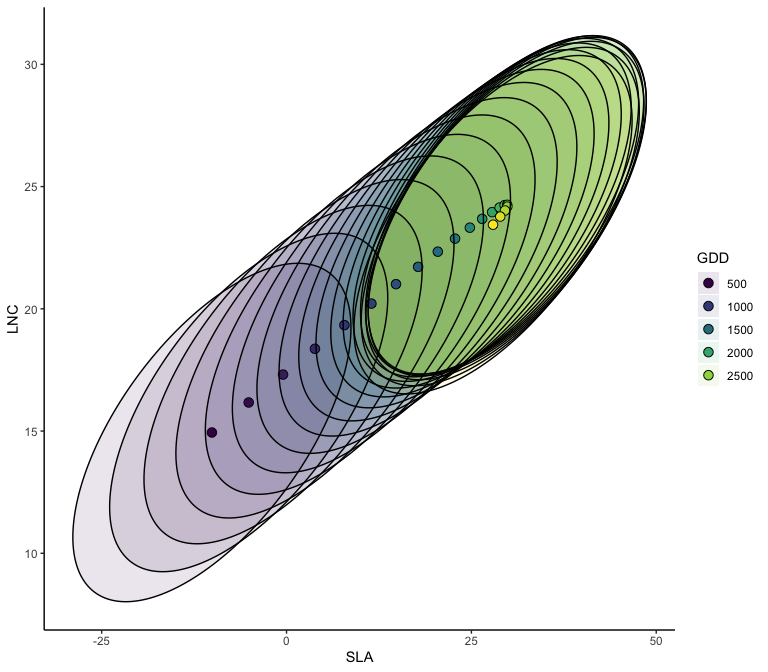The user can also choose to fix the non-focal environmental variables to another value. For example, we can obtain the partial response curves of the most likely CWM combination and envelop of possible CWM combinations of SLA and GDD in forest. # plot the pairwise SLA-LNC partial response curve along the GDD gradient ellipse_plot(m, indexTrait = c("SLA","LNC"), indexGradient="GDD", FixX = list(GDD = NULL, FDD = NULL, forest = 1))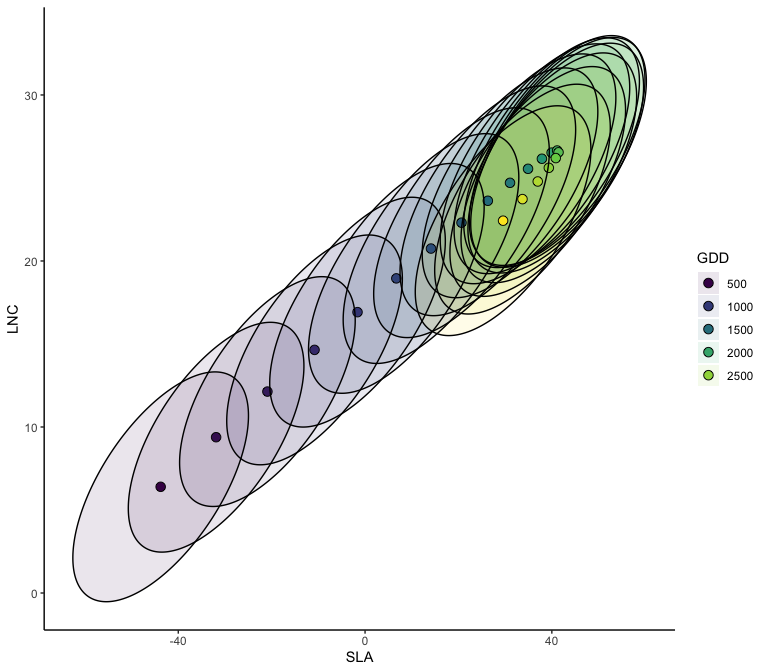## Joint predictions The jtdm package allows to define a region in the community-trait space and compute their joint probabilities for a given set of environmental conditions. This is done by the function joint_trait_prob. To define a given region in the community-trait space, the user has to define the focal traits indexTrait (any number of traits is accepted) and trait-specific thresholds through the parameter bounds. bounds is a list of the length of indexTrait, where each element of the list is a vector of length two. The vector represents the inferior and superior bounds of the region for the specified trait. For example, if we consider two traits (e.g. SLA and LNC), bounds=list(c(20,Inf),c(20,Inf)) corresponds to the region in the community-trait space where both SLA and LNC both take values greater than 20. We can then define the sites (i.e. the set of environmental conditions) in which to compute joint probabilities. For example we can compute joint probabilities of both SLA and LNC to be greater than 20 in a high altitude site. This measures the relative suitability of communities where both SLA and LNC are higher than 20 in a high altitude site. joint_trait_prob(m, indexTrait = c("SLA","LNC"), Xnew = X["VCHA_2940",], bounds = list(c(20,Inf),c(20,Inf)), FullPost = TRUE)$PROBmean
#>          1
#> 0.09324214

Unsurprisingly, the probability is low. Then, we compute how this probability vary along the GDD gradient using the function joint_trait_prob_gradient. The function builts a dataframe where the focal variable varies along a gradient and the other (non-focal) variables are fixed to their mean (but see FixX parameter for fixing non-focal variables to user-defined values) and predict the joint probabilities along the gradient.

joint = joint_trait_prob_gradient(m, indexTrait = c("SLA","LNC"),
bounds = list(c(mean(Y[,"SLA"]),Inf),c(mean(Y[,"SLA"]),Inf)),
FullPost = TRUE)

We can then plot such predictions.

#> Warning: Using size aesthetic for lines was deprecated in ggplot2 3.4.0.
#> ℹ Please use linewidth instead.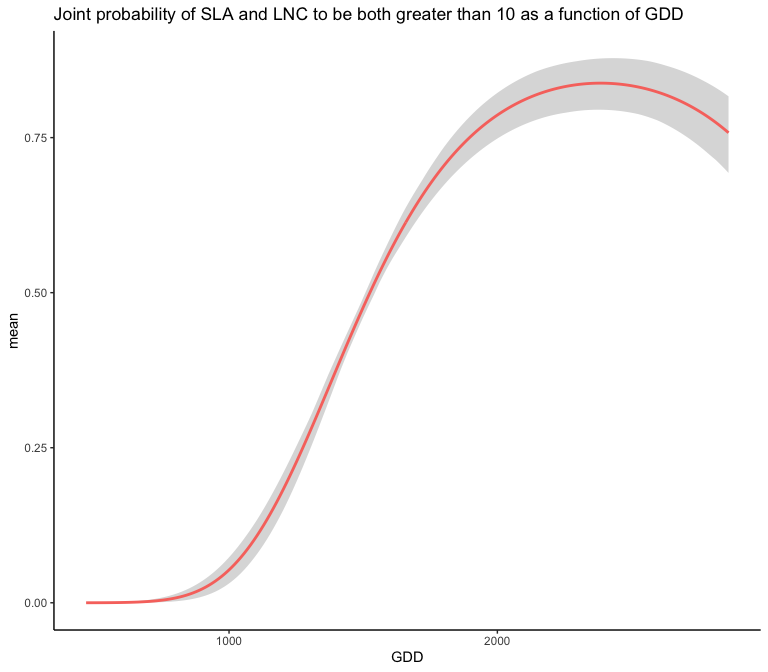As climatic conditions become more favorable (i.e. GDD increases), the probability of having high values of both traits increases.

# Author

This package is currently developed by Giovanni Poggiato from Laboratoire d’Ecologie Alpine. It is supported by the ANR GAMBAS. The framework implemented in this package is described in: Joint modeling and predictions of community traits. Poggiato Giovanni, Gaüzere Pierre, Martinez Almoyna, Camille, Deschamps, Gabrielle, Renaud, Julien, Violle, Cyrille, Münkemüller, Tamara, Thuiller, Wilfried. In preparation.